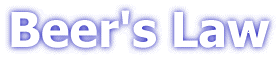### Introduction

Many compounds absorb ultraviolet (UV) or visible (Vis.) light. The diagram below shows a beam of monochromatic radiation of radiant power P0, directed at a sample solution. Absorption takes place and the beam of radiation leaving the sample has radiant power P.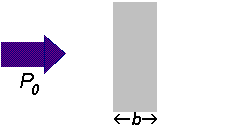The amount of radiation absorbed may be measured in a number of ways: Transmittance, T = P / P0 % Transmittance, %T = 100 T Absorbance, A = log10 P0 / P A = log10 1 / T A = log10 100 / %T A = 2 - log10 %T

The last equation, A = 2 - log10 %T , is worth remembering because it allows you to easily calculate absorbance from percentage transmittance data.

The relationship between absorbance and transmittance is illustrated in the following diagram: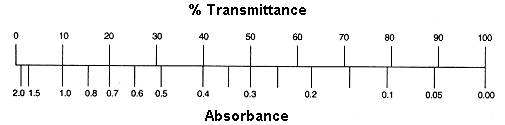So, if all the light passes through a solution without any absorption, then absorbance is zero, and percent transmittance is 100%. If all the light is absorbed, then percent transmittance is zero, and absorption is infinite.

### The Beer-Lambert Law

Now let us look at the Beer-Lambert law and explore it's significance. This is important because people who use the law often don't understand it - even though the equation representing the law is so straightforward:

A=ebc

Where A is absorbance (no units, since A = log10 P0 / P )
e
is the molar absorbtivity with units of L mol-1 cm-1
b is the path length of the sample - that is, the path length of the cuvette in which the sample is contained. We will express this measurement in centimetres.
c is the concentration of the compound in solution, expressed in mol L-1

The reason why we prefer to express the law with this equation is because absorbance is directly proportional to the other parameters, as long as the law is obeyed. We are not going to deal with deviations from the law.

Let's have a look at a few questions...

Question : Why do we prefer to express the Beer-Lambert law using absorbance as a measure of the absorption rather than %T ?

A=ebc

%T = 100 P/P0 = e -ebc

Now, suppose we have a solution of copper sulphate (which appears blue because it has an absorption maximum at 600 nm). We look at the way in which the intensity of the light (radiant power) changes as it passes through the solution in a 1 cm cuvette. We will look at the reduction every 0.2 cm as shown in the diagram below. The Law says that the fraction of the light absorbed by each layer of solution is the same. For our illustration, we will suppose that this fraction is 0.5 for each 0.2 cm "layer" and calculate the following data:

 Path length / cm 0 0.2 0.4 0.6 0.8 1 %T 100 50 25 12.5 6.25 3.125 Absorbance 0 0.3 0.6 0.9 1.2 1.5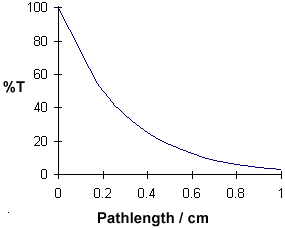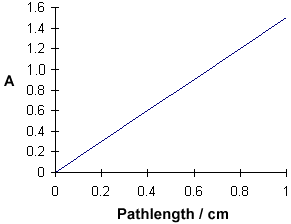A = ebc tells us that absorbance depends on the total quantity of the absorbing compound in the light path through the cuvette. If we plot absorbance against concentration, we get a straight line passing through the origin (0,0).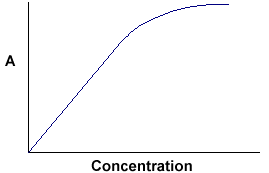Note that the Law is not obeyed at high concentrations. This deviation from the Law is not dealt with here.

The linear relationship between concentration and absorbance is both simple and straightforward, which is why we prefer to express the Beer-Lambert law using absorbance as a measure of the absorption rather than %T.

Question : What is the significance of the molar absorbtivity, e ?

Answer : To begin we will rearrange the equation A = ebc :

e = A / bc

In words, this relationship can be stated as "e is a measure of the amount of light absorbed per unit concentration".

Molar absorbtivity is a constant for a particular substance, so if the concentration of the solution is halved so is the absorbance, which is exactly what you would expect.

Let us take a compound with a very high value of molar absorbtivity, say 100,000 L mol-1 cm-1, which is in a solution in a 1 cm pathlength cuvette and gives an absorbance of 1.

e = 1 / 1 ´ c

Therefore, c = 1 / 100,000 = 1 ´ 10-5 mol L-1

Now let us take a compound with a very low value of e, say 20 L mol-1 cm-1 which is in solution in a 1 cm pathlength cuvette and gives an absorbance of 1.

e = 1 / 1 ´ c

Therefore, c = 1 / 20 = 0.05 mol L-1

The answer is now obvious - a compound with a high molar absorbtivity is very effective at absorbing light (of the appropriate wavelength), and hence low concentrations of a compound with a high molar absorbtivity can be easily detected.

Question : What is the molar absorbtivity of Cu2+ ions in an aqueous solution of CuSO4 ? It is either 20 or 100,000 L mol-1 cm-1

Answer : I am guessing that you think the higher value is correct, because copper sulphate solutions you have seen are usually a beautiful bright blue colour. However, the actual molar absorbtivity value is 20 L mol-1 cm-1 ! The bright blue colour is seen because the concentration of the solution is very high.

b-carotene is an organic compound found in vegatables and is responsible for the colour of carrots. It is found at exceedingly low concentrations. You may not be surprised to learn that the molar absorbtivity of b-carotene is 100,000 L mol-1 cm-1 !

You should now have a good understanding of the Beer-Lambert Law; the different ways in which we can report absorption, and how they relate to each other. You should also understand the importance of molar absorbtivity, and how this affects the limit of detection of a particular compound.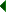Back to index of topics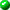Beer's LawBeer's Law - Quiz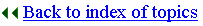Biosciences Homepage